With these tools you can very quickly calculate one of the underlying functions using a simple data entry method.

The calculators in this section are practical tools that have informational value and do not constitute advice and do not represent express or implied guarantees.

## Design Tools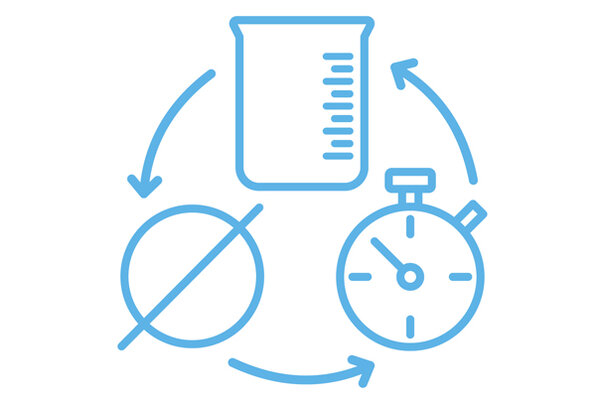This calculation tool measures the inside diameter of a pipe, flow rate or velocity. Entering two of the three required parameters automatically calculates the third.The purpose of this calculation tool is to find the volume and time it takes to empty a tank, knowing the shape, size and height of the liquid it contains.This calculation tool allows you to calculate the power absorbed by a pump knowing the characteristics of the pipe and the fluid, the pressures and the pressure drop. The values ​​of some parameters such as the efficiency of the pump, the density of the liquid and the roughness of the pipe are already suggested as they are very common, but obviously they can be modified in case of different operating conditions.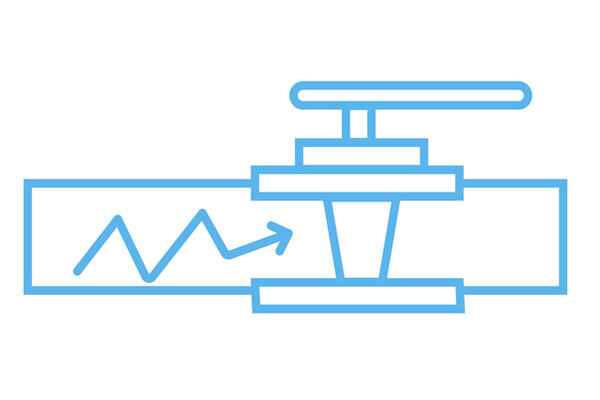Water hammer is a transient increase in pressure when flow stops or begins rapidly. This tool calculates maximum pressure based on pipe characteristics, valve closure time, liquid velocity and inlet pressure.The purpose of this calculation tool is to measure the flow rate in a valve by knowing its flow coefficient, the difference between the outlet and inlet pressures and the specific gravity of the liquid compared to water at 15°C.This calculator measures a valve's flow coefficient by knowing its flow rate, the difference between outlet and inlet pressures, and the specific gravity of the fluid compared to that of water at 15°C.This calculation tool allows you to measure the pressure difference across a valve by knowing the flow coefficient, flow rate and specific gravity of the liquid compared to water at 15°C.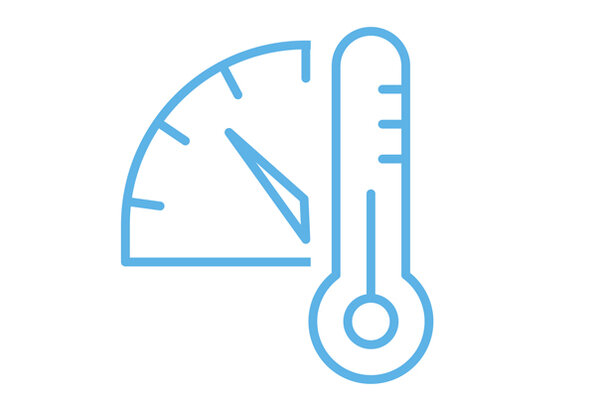This tool plots the relationship between nominal pressure and temperature based on the type of material used and the SDR. The graph obtained has been calculated considering a 25 year service life.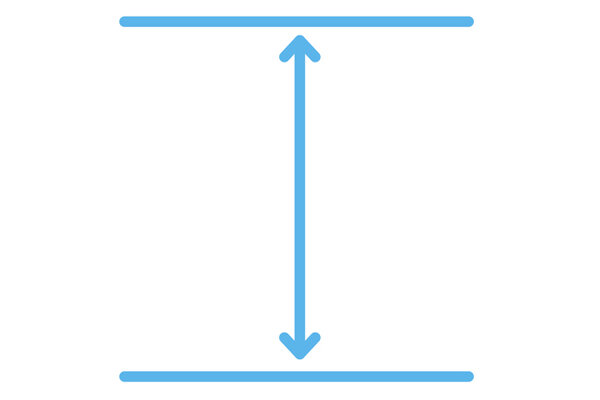Flow Rate Calculation Based on the Size of the Valve Based on the operating conditions of the process, this tool allows you to calculate the flow rate of the fluid that can flow inside the valve in use. By entering the selected valve type, the inlet and outlet pressure and the fluid properties as input, the tool displays the characteristic curve diagram. In this way it is possible to identify the flow range in which operation is recommended, depending on the degree of opening of the valve. In order to achieve optimal regulation, it is recommended to size the valve so that the flow remains in the middle of the curves.With this calculation tool you can calculate the total pressure losses within a system, i. H. distributed and local pressure drops. Distributed pressure drops occur when the energy loss is generated by the surface friction developed between the fluid and the tube walls. In the case of point leaks, on the other hand, the energy loss is generated by form friction, due to a change in direction of the fluid in the presence of valves or fittings.

## Tools for installationPipes can expand and contract due to temperature changes in a system. To prevent breakage of fasteners, compensators are usually used, or expansion rings or changes in direction are made. This tool allows us to calculate the right parameters to prevent the potential expansion or contraction of our systems.Pipes can expand and contract due to temperature changes in a system. To prevent breakage of fasteners, compensators are usually used, or expansion rings or changes in direction are made. This tool allows us to calculate the right parameters to prevent the potential expansion or contraction of our systems.With this calculation tool it is possible to define the minimum distance between the supports of a pipe according to the characteristics of the pipe itself, the temperature and the liquid flowing in it.This calculator gives the most important parameters for butt welding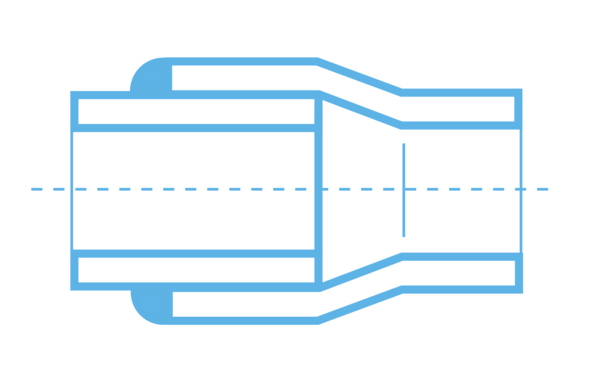This calculator gives the most important parameters for socket welding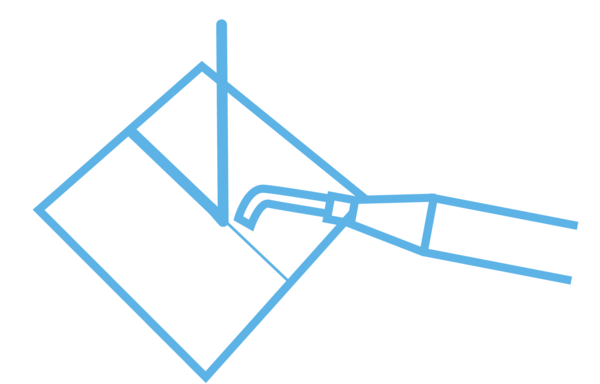This calculator shows the most important parameters for hot gas welding